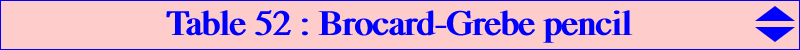The second Brocard cubic K018 and the Grebe cubic K102 are both isogonal cubics, the former being a nK0 and the latter a pK. They generate a pencil of K0s passing through the base points A, B, C, G = X(2), K = X(6) and the four foci of the inconic with center X(6). Any such cubic Γ(k) of the pencil has an equation of the form : ∑ (y - z) [a^4 y z + b^2 c^2 x (k x + y + z)] = 0, where k is a real number or infinity. Properties • the pencil is stable under isogonal conjugation and the isogonal transform of Γ(k) is Γ(k)* = Γ(–k). It follows that K018 = Γ(0) and K102 = Γ(∞) are the only self-isogonal cubics of the pencil. • Γ(k) meets the line GK = X(2)X(6) again at P(k) = b^2 + c^2 - (2 + k) a^2 : : , the homothetic of G under h(K, - 3/k). • Γ(k) meets the line at infinity at the same points as pK(X6, P(-k)) and the circumcircle at the same points as pK(X6, P(k)). Obviously, P(0) = X(524) and P(∞) = X(6). • Γ(k) is spK(P(-k), X6) in CL055. • the pencil contains two other pKs, two other (decomposed) nK0s, two K+ and one K++ but no K60. These are shown in the table below.k P cubic / other centers X(i) on the cubic for i = type remarks/ notes ∞ X(6) K102 / 1, 43, 87, 194, 3224 pK (7) - k1 X(385) K757 / 182, 264, 287, 511, 694 k1 = 1 - 4 sin2ω -3 GK/R ? - / 1114, 1344, 2574 nK0 decomposed -3 X(2) K1093 isogonal transform of K314 / 21448 spK(X1992, X6) (1) -2 X(141) K754 / 17, 18, 141, 9481 spK(X3629, X6) (3) -1 X(69) K168 / 3, 69, 485, 486, 5374, 5408, 5409, 6337, 8770 pK (4) 0 X(524) K018 / 13, 14, 15, 16, 111, 368, 524, 5000, 5001 nK0 focal cubic, (6) 1 X(193) K233 / 4, 25, 193, 371, 372, 2362, 7133 pK (5) 2 X(3629) K755 / 61, 62, 251, 3629, 9484 spK(X141, X6) (2) 3 X(1992) K314 / 1992 K++, spK(X2, X6) central cubic 3 GK/R ? - / 1113, 1345, 2575 nK0 decomposed k1 X(39099) K756 / 98, 184, 232, 262, 385, 7709 k1 = 1 - 4 sin2ω (3 - k2)/2 ? - / K+ k2 below (3 + k2)/2 ? - / K+k2 is the square root of : 25 + 16 cosA cosB cosCSpecial points on these cubics(1) : vertices of the Thomson triangle (2) : Ix-anticevian points, see Table 23 (3) : isogonal conjugates of the Ix-anticevian points, see Table 23 (4) : H-cevian points, see Table 11 (5) : isogonal conjugates of the H-cevian points, see Table 11 (6) : four foci of the Steiner inellipse (7) : imaginary foci of the Brocard inellipse